# High School Physics : Understanding Accuracy and Precision

## Example Questions

### Example Question #1 : Understanding Accuracy And Precision

Michael buys several bags of balloons. On the package, it says that each bag has 100 balloons. He opens the bags and only one of them has 100 balloons inside; the other bags either have too many or too few.

How would you describe the bag of balloons with 100 balloons inside?

Precise, but not accurate

Neither accurate nor precise

Accurate, but the precision cannot be determined

Accurate, but not precise

Both accurate and precise

Accurate, but not precise

Explanation:

This bag is accurate because it provided the correct number of balloons, however, the process is not precise as the results were clearly not repeatable.

Accuracy deals with how close the measurement got to the accepted measurement. Precision deals with how consistent the measurement is. The bag with 100 balloons inside matched the claim made on the bag, meaning it was accurate. It was not precise because the other measurements show that the number of balloons is variable.

### Example Question #2 : Understanding Accuracy And Precision

Michael's scale measures the mass of objects as consistently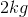less than their actual mass. How would you describe the scale?

It is accurate, but not precise

It is neither accurate nor precise

It is precise, but not accurate

Accuracy and precision are synonyms

It is both accurate and precise

It is precise, but not accurate

Explanation:

Precision measures is how consistently a device records the same answer. In this case, Michael's scale is ALWAYSshort. Even though it displays the wrong value, it is consistent. That means it is precise. Measuring a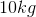object will always display a mass of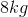; the results are easily reproduced.

Accuracy is how well a device measures something against its accepted value. In this case, Michael's scale is not accurate because it is always off by.

### Example Question #3 : Understanding Accuracy And Precision

An brand of fruit snacks claims that each bag of fruit snacks has a mass of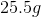. After weighing three bags, Wally observes the masses to be,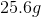, and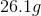.

How can Wally describe the accuracy and precision of the first bag he measured?

Accurate, but not precise

Neither accurate nor precise

Accurate and precise

There are insufficient data points to draw a conclusion

Precise, but not accurate

Accurate, but not precise

Explanation:

The claim for the mass of the first bag is accurate; the brand says there should bein each bag and there wasin the first bag.

The claim on the first bag is not precise, as the results are not replicated universally throughout the experiment. The masses of the bags fluctuate, with the average of all three bags equal to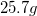.

### Example Question #4 : Understanding Accuracy And Precision

A bowman is shooting arrows at a target. Which of the following demonstrates high accuracy but low precision?

The bowman consistently misses the target and hits a tree in the same spot

The bowman consistently hits around the target but never hits the bullseye

The bowman consistently hits the bullseye

The bowman consistently hits to the left of the target

The bowman consistently hits to the right of the bullseye

The bowman consistently hits around the target but never hits the bullseye

Explanation:

Accuracy is measured as the degree of closeness to the actual measurement. In our case, accurate shots will hit the bullseye. Precision is measured as the degree of closeness of one measurement to the next. In our case, precise shots will be clustered together.

To get high accuracy but low precision, measurements must center around the target value but be variable. The bowman's arrows will not be clustered (low precision), but will be accurately distributed around the bullseye. If all the shots were averaged, the bullseye would be at the center.

### Example Question #5 : Understanding Accuracy And Precision

Which of these is an example of high precision?

A student tries to throw a pencil into the garbage can and makes it in

A student correctly calculates the mass of an object to be 54kg

A student correctly calculates the acceleration due to gravity to be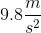An archer hits the bulls-eye

An archer hits the same spot on the target three times in a row

An archer hits the same spot on the target three times in a row

Explanation:

Precision is a measure of reproducibility. If multiple trials produce the same result each time with minimal deviation, then the experiment has high precision. This is true even if the results are not true to the theoretical predictions; an experiment can have high precision with low accuracy.

In contrast, accuracy is the measure of difference between a calculated value and the true value of a measurement. High accuracy demands that the experimental result be equal to the theoretical result.

An archer hitting a bulls-eye is an example of high accuracy, while an archer hitting the same spot on the bulls-eye three times would be an example of high precision.

### Example Question #3 : Understanding Accuracy And Precision

Which of these is an example of high accuracy?

An archer hits the bulls-eye

A student calculates the acceleration due to gravity to be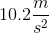using three different methods

A student throws three pencils into the trash can, but misses and hits the window each time

An archer hits the same spot on the target three times in a row

A student repeatedly calculates the mass of an object to be 49 grams, when the true mass is 52 grams